## Summary

So far we only used the vertex shader to move vertices from their object coordinates to their clip space coordinates (or to the world space coordinates which we then used for other things). But there are more things we can do with vertex shaders. As a introduction I’m going to show you how to apply a simple sine wave to a model, making it wobble.

I will make the shader with a surface shader so you should know the basics of surface shaders, but it works the same with any other type of shader.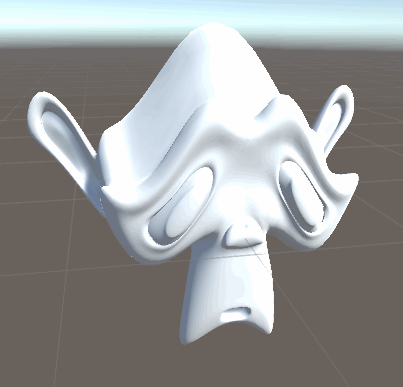When manipulating the positions of our surface, we use the vertex shader. So far we didn’t write a vertex shader in our surface shader, it was instead generated by unity in the background. To change that we add the declaration for it in our surface shader definition by adding the vertex:vertexShaderName part.

``````//the shader is a surface shader, meaning that it will be extended by unity in the background
//to have fancy lighting and other features
//our surface shader function is called surf and we use our custom lighting model
//fullforwardshadows makes sure unity adds the shadow passes the shader might need
//vertex:vert makes the shader use vert as a vertex shader function
#pragma surface surf Standard fullforwardshadows vertex:vert
``````

Then we have to write the actual vertex function. Previously, in unlit shaders, we calculated the clip space position in there, but even when using vertex shaders, that part is generated for us in surface shaders. We manipulate the object space vertex positions and then let them be processed by unity.

Because the input struct has to have variables with specific names, it’s easiest to use a input struct unity provides for us here, it’s called appadata_full, but we could also use our own struct here if it uses the same terminology.

Just like the surface shader, the vertex shader in surface shaders (there should be better terminology for this) doesn’t return anything, instead it takes a parameter with the inout keyword we can manipulate.

Because surface shaders generate the conversion to clip space for us, a empty vertex function is all we need to make our shader work just like before.

``````void vert(inout appdata_full data){

}
``````

A simple thing we can do to our mesh is multiply all of our vertices by a value to make the model bigger. (a *= b is the same as a = a * b but a bit shorter)

``````void vert(inout appdata_full data){
data.vertex.xyz *= 2;
}
``````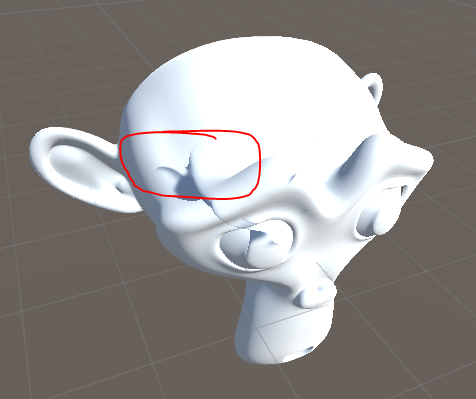While the model is bigger we also see a weird artefact here. The shadow is still calculated based on the original, unmodified vertex positions. That’s because the surface shader doesn’t automatically generate a shadow pass (used for casting shadows) for our new vertex positions. To fix that we expand our surface definition with the hint addshadows and the artefects should be gone.

``````//addshadows tells the surface shader to generate a new shadow pass based on out vertex shader
#pragma surface surf Standard fullforwardshadows vertex:vert addshadow
``````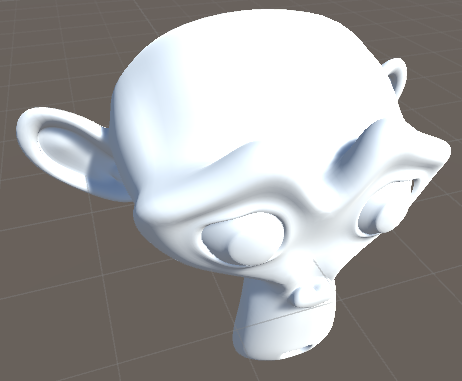To make the shader more interresting we’ll change the vertex shader. Instead of making the model just bigger, we’ll offset the y position based on the sine of the x position, making it wavy.

``````void vert(inout appdata_full data){
data.vertex.y += sin(data.vertex.x);
}
``````This results in big waves with a low frequency, so we’ll add two variables to change those properties.

``````//...

_Amplitude ("Wave Size", Range(0,1)) = 0.4
_Frequency ("Wave Freqency", Range(1, 8)) = 2

//...

float _Amplitude;
float _Frequency;

//...

void vert(inout appdata_full data){
float4 modifiedPos = data.vertex;
modifiedPos.y += sin(data.vertex.x * _Frequency) * _Amplitude;
data.vertex = modifiedPos;

//...
``````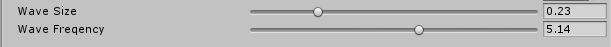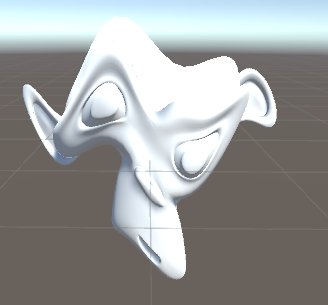With this we have nice customizable waves on our model, but sadly the normals of our deformed models are wrong. We only moved the positions, not the normals.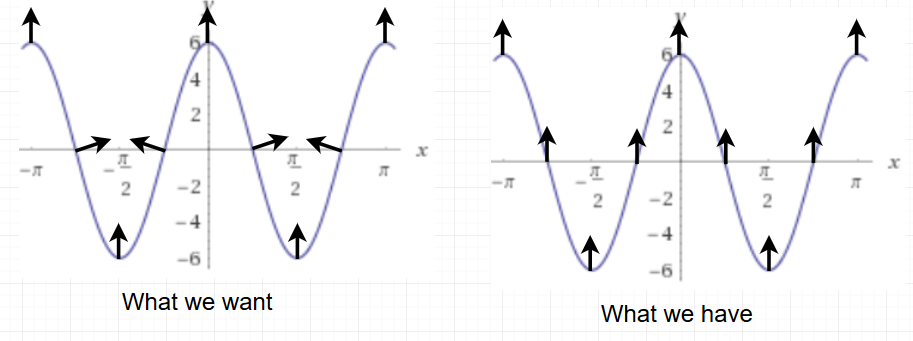The easiest and most flexible way to generate correct normals for custom geometry is to calculate the custom geometry for neighboring surface points and recalculate the normal from that.

To get neighboring surface points we can follow the tangent and bitangent of the surface. The normal, the tangent and the bitangent are all orthogonal to each other. The tangent and the bitangent both lie on the surface of the object.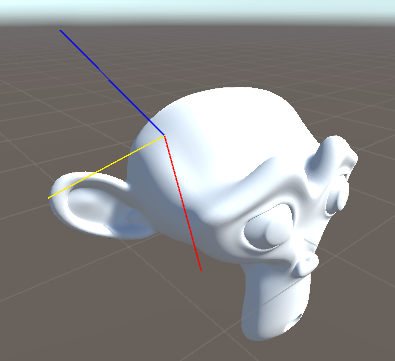Normal in blue, tangent in red and bitangent in yellow.

Luckily the tangent are already saved in the model data, so we can just use them. The bitangent isn’t, but we can calculate it easily by taking the cross product of the normal and the tangent (taking the cross product of two vectors returns a vector that’s orthogonal to both).

After we obtain the bitangent we create two new points that are almost at the vertex position, but slightly changed, and give them the same treatment we gave the original position.

``````float3 posPlusTangent = data.vertex + data.tangent * 0.01;
posPlusTangent.y += sin(posPlusTangent.x * _Frequency) * _Amplitude;

float3 bitangent = cross(data.normal, data.tangent);
float3 posPlusBitangent = data.vertex + bitangent * 0.01;
posPlusBitangent.y += sin(posPlusBitangent.x * _Frequency) * _Amplitude;
``````

With those positions we can now calculate the new normal of the surface. For that we calculate a new tangent and bitangent from the positions by subtracting the modified base surface position from the modified surface positions where we added the tangent/bitangent previously. And after obtaining the new tangent and bitangent, we can take their cross product to get the new normal which we then use.

``````void vert(inout appdata_full data){
float4 modifiedPos = data.vertex;
modifiedPos.y += sin(data.vertex.x * _Frequency) * _Amplitude;

float3 posPlusTangent = data.vertex + data.tangent * 0.01;
posPlusTangent.y += sin(posPlusTangent.x * _Frequency) * _Amplitude;

float3 bitangent = cross(data.normal, data.tangent);
float3 posPlusBitangent = data.vertex + bitangent * 0.01;
posPlusBitangent.y += sin(posPlusBitangent.x * _Frequency) * _Amplitude;

float3 modifiedTangent = posPlusTangent - modifiedPos;
float3 modifiedBitangent = posPlusBitangent - modifiedPos;

float3 modifiedNormal = cross(modifiedTangent, modifiedBitangent);
data.normal = normalize(modifiedNormal);
data.vertex = modifiedPos;
}
``````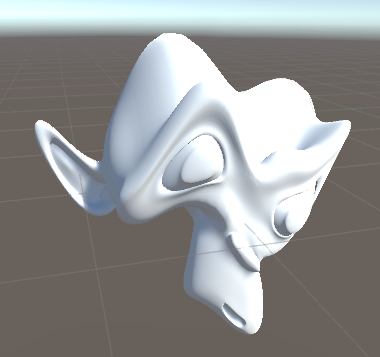The last thing I’d like to add to this shader is movement over time. So far we only use the x position of the vertex as a changing parameter in our function which generates the new vertex positions, but adding the time to that is pretty easy.

Unity passes the time to all shaders automatically as a 4-dimentional vector, the first component of the vector is the time divided by 20, the second just the time in seconds, the third the time multiplied by 2 and the fourth contains the time multiplied by 3. Because we want to adjust the time via a external property we use the second component, with the time in seconds. We then add the time multiplied by the animation speed to the x position.

``````_AnimationSpeed ("Animation Speed", Range(0,5)) = 1

//...

float _AnimationSpeed;

//...

void vert(inout appdata_full data){
float4 modifiedPos = data.vertex;
modifiedPos.y += sin(data.vertex.x * _Frequency + _Time.y * _AnimationSpeed) * _Amplitude;

float3 posPlusTangent = data.vertex + data.tangent * 0.01;
posPlusTangent.y += sin(posPlusTangent.x * _Frequency + _Time.y * _AnimationSpeed) * _Amplitude;

float3 bitangent = cross(data.normal, data.tangent);
float3 posPlusBitangent = data.vertex + bitangent * 0.01;
posPlusBitangent.y += sin(posPlusBitangent.x * _Frequency + _Time.y * _AnimationSpeed) * _Amplitude;

float3 modifiedTangent = posPlusTangent - modifiedPos;
float3 modifiedBitangent = posPlusBitangent - modifiedPos;

float3 modifiedNormal = cross(modifiedTangent, modifiedBitangent);
data.normal = normalize(modifiedNormal);
data.vertex = modifiedPos;
}
``````I increased the offset of the sampled surface positions a bit (up to 0.01 units) to smooth over the artefacts better. A small distance can represent a more complex distortion better while bigger distances smoothes over some things.

``````Shader "Tutorial/015_vertex_manipulation" {
//show values to edit in inspector
Properties {
_Color ("Tint", Color) = (0, 0, 0, 1)
_MainTex ("Texture", 2D) = "white" {}
_Smoothness ("Smoothness", Range(0, 1)) = 0
_Metallic ("Metalness", Range(0, 1)) = 0
[HDR] _Emission ("Emission", color) = (0,0,0)

_Amplitude ("Wave Size", Range(0,1)) = 0.4
_Frequency ("Wave Freqency", Range(1, 8)) = 2
_AnimationSpeed ("Animation Speed", Range(0,5)) = 1
}
SubShader {
//the material is completely non-transparent and is rendered at the same time as the other opaque geometry
Tags{ "RenderType"="Opaque" "Queue"="Geometry"}

CGPROGRAM

//the shader is a surface shader, meaning that it will be extended by unity in the background
//to have fancy lighting and other features
//our surface shader function is called surf and we use our custom lighting model
//fullforwardshadows makes sure unity adds the shadow passes the shader might need
//vertex:vert makes the shader use vert as a vertex shader function
//addshadows tells the surface shader to generate a new shadow pass based on out vertex shader
#pragma surface surf Standard fullforwardshadows vertex:vert addshadow
#pragma target 3.0

sampler2D _MainTex;
fixed4 _Color;

half _Smoothness;
half _Metallic;
half3 _Emission;

float _Amplitude;
float _Frequency;
float _AnimationSpeed;

//input struct which is automatically filled by unity
struct Input {
float2 uv_MainTex;
};

void vert(inout appdata_full data){
float4 modifiedPos = data.vertex;
modifiedPos.y += sin(data.vertex.x * _Frequency + _Time.y * _AnimationSpeed) * _Amplitude;

float3 posPlusTangent = data.vertex + data.tangent * 0.01;
posPlusTangent.y += sin(posPlusTangent.x * _Frequency + _Time.y * _AnimationSpeed) * _Amplitude;

float3 bitangent = cross(data.normal, data.tangent);
float3 posPlusBitangent = data.vertex + bitangent * 0.01;
posPlusBitangent.y += sin(posPlusBitangent.x * _Frequency + _Time.y * _AnimationSpeed) * _Amplitude;

float3 modifiedTangent = posPlusTangent - modifiedPos;
float3 modifiedBitangent = posPlusBitangent - modifiedPos;

float3 modifiedNormal = cross(modifiedTangent, modifiedBitangent);
data.normal = normalize(modifiedNormal);
data.vertex = modifiedPos;
}

//the surface shader function which sets parameters the lighting function then uses
void surf (Input i, inout SurfaceOutputStandard o) {
//sample and tint albedo texture
fixed4 col = tex2D(_MainTex, i.uv_MainTex);
col *= _Color;
o.Albedo = col.rgb;
//just apply the values for metalness, smoothness and emission
o.Metallic = _Metallic;
o.Smoothness = _Smoothness;
o.Emission = _Emission;
}
ENDCG
}
FallBack "Standard"
}
``````

You can also find the source code for this tutorial here: https://github.com/ronja-tutorials/ShaderTutorials/blob/master/Assets/015_VertexManipulation/vertexmanipulation.shader

I hope I was able to explain how to start manipulating vertices and you find your own ways of making nice looking shaders with this technique.

You can also find me on twitter at @totallyRonja. If you liked my tutorial and want to support me you can do that on Patreon (patreon.com/RonjaTutorials) or Ko-Fi (ko-fi.com/RonjaTutorials).